# Assignment 4

This notebook looks at the unemployment rate for the Portland-Hillsboro-Vancouver area (in Oregon and Washington State) from January 2007 through February 2017. I want to answer the questions - How has Portland's unemployment rate changed over the last ten years and how does it compare to the national unemployment rate? Additionally, I'd like to see how well this metric follows the S&P 500 Index and the Housing Price Index.

## Imports and Setup

# python standard library
import os

# third-party
import matplotlib.pyplot as pyplot
import numpy
import pandas
import pygal
import requests
import seaborn

% matplotlib inline
seaborn.set_style("whitegrid")


## Data

### Constants

class Urls(object):
national = "https://www.dropbox.com/s/qw2l5hmu061l8x2/national_unemployment.csv?dl=1"
portland = "https://www.dropbox.com/s/wvux3d7dcaae5t0/portland_unemployment_2007_2017.csv?dl=1"
house_price = "https://www.dropbox.com/s/4hu2jpjkhcnr35k/purchase_only_house_price_index.csv?dl=1"
s_and_p = "https://www.dropbox.com/s/ojj5zp7feid6wwl/SP500_index.csv?dl=1"

class Paths(object):
portland = "portland_unemployment_2007_2017.csv"
national = "national_unemployment.csv"
s_and_p = "SP500_index.csv"
house_price = "purchase_only_house_price_index.csv"


def download_data(path, url):
Args:
path (str): path to the file

"""
if not os.path.isfile(path):
response = requests.get(url)
with open(path, 'w') as writer:
writer.write(response.text)
return


## Portland

The data represents Local Area Unemployment Statistics for the Portland-Hillsboro-Vancouver area in Oregon and was taken from the Bureau of Labor Statistics (the Portland-Vancouver-Hillsboro, OR-WA Metropolitan Statistical Area). It has monthly values starting from January 2007 and continues through February 2017.

Data extracted on: Apr 30, 2017 (6:54:00 PM)

Local Area Unemployment Statistics

Series Title : Unemployment Rate: Portland-Vancouver-Hillsboro, OR-WA Metropolitan Statistical Area (U) Series ID : LAUMT413890000000003 Seasonality : Not Seasonally Adjusted Survey Name : Local Area Unemployment Statistics Measure Data Type : unemployment rate Area : Portland-Vancouver-Hillsboro, OR-WA Metropolitan Statistical Area Area Type : Metropolitan areas

download_data(Paths.portland, Urls.portland)

portland.describe()

portland.head()


### Cleaning

I changed to a slightly different data-source so that I could get direct links to the data, so I'm going to re-name some of the columns to match what I was using befroe

column_renames = {"Value": "unemployment_rate",
"Label": "date"}
portland.rename(columns=column_renames,
inplace=True)
portland.columns


Now I'll re-do the dates.

portland.Period.unique()


I use the months in one of the plots as labels so I'm going to create a column with just their (abbreviated) names.

month_map = dict(M01="Jan", M02="Feb", M03="Mar", M04="Apr", M05="May",
M06="Jun", M07="Jul", M08="Aug", M09="Sep", M10="Oct",
M11="Nov", M12="Dec")
portland["month"] = portland.Period.apply(lambda x: month_map[x])


In the plot I'm going to mark where the unemployment was at its highest point.

highest_unemployment = portland.unemployment_rate.max()
print(highest_unemployment)
unemployment_peaks = numpy.where(portland.unemployment_rate==highest_unemployment)

unemployment_peaks

print(portland.date.ix[unemployment_peaks])
print(portland.date.ix[unemployment_peaks])


It looks like it reached 11.4% twice - on June, 2009 and January of 2010.

lowest_unemployment = portland.unemployment_rate.min()
print(lowest_unemployment)
print(highest_unemployment/lowest_unemployment)
print(str(portland.date.ix[numpy.where(
portland.unemployment_rate==lowest_unemployment)]))


At its peak, the unemployment rate for the Portland-Hillsboro-Vancouver area was almost three times higher than the most recent (preliminary) unemployment rate.

According to the National Bureau of Economic Research, the most recent economic contraction occurred from December 2007 through June 2009 which falls within the data set so I'll highlight that on the plot.

recession_start = numpy.where(portland.date=="2007 Dec")
recession_end = numpy.where(portland.date=="2009 Jun")
portland_recession_start = portland.unemployment_rate.iloc[recession_start]
print(portland_recession_start)
print(portland.unemployment_rate.iloc[recession_end])


When did it reach the recession-start rate?

portland.date.iloc[numpy.where(portland.unemployment_rate==portland_recession_start)]


### Unemployment Rate Over Time

First I'll plot how the unemployment rate changed over time.

figure = pyplot.figure(figsize=(10, 10))
axe = figure.gca()
seaborn.set_style("whitegrid")
portland.plot(x="date", y="unemployment_rate", ax=axe, legend=False)
axe.set_title("Portland-Hillsboro-Vancouver Unemployment Over Time")
axe.set_ylabel("% Unemployed")
axe.set_xlabel("Month")
seaborn.despine()


It looks like unemployment was relatively low until September of 2008, when it suddenly spiked before beginning a steady downward trend.

## National

As a comparison, I downloaded the unemployment rate data for the nation as a whole (also taken from the Bureau of Labor Statistics.

NATIONAL_PATH = "national_unemployment.csv"
NATIONAL_URL = "https://www.dropbox.com/s/qw2l5hmu061l8x2/national_unemployment.csv?dl=1"

national.rename(columns=column_renames, inplace=True)


The local data has one fewer month than the national one so I'll remove it here.

national.tail()

national.drop(, inplace=True)
national.tail()

peak = national.unemployment_rate.max()
print(peak)
national_peak = numpy.where(national.unemployment_rate==peak)
print(portland.date.iloc[national_peak])


When did it reach the same level it was at when the recession began?

national_recession_start = national.unemployment_rate.iloc[recession_start]
post_recession = national[national.Year > 2009]
index = numpy.where(post_recession.unemployment_rate==national_recession_start)
post_recession.date.iloc[index]


### Plotting

I'm not going to be looking at the numbers so much as comparing plots from now on so I'll remove the grid.

style = seaborn.axes_style("whitegrid")
style["axes.grid"] = False
seaborn.set_style("whitegrid", style)

figure = pyplot.figure(figsize=(10, 10))
axe = figure.gca()
national.plot(x="date", y="unemployment_rate", ax=axe, legend=False)
portland.plot(x="date", y="unemployment_rate", ax=axe, legend=False)
axe.set_ylabel("% Unemployment")
axe.set_title("Unemployment Rate (Jan 2007 - Feb 2017)")

last = portland.date.count()
axe.text(last, national["unemployment_rate"].iloc[-1], "National")
axe.text(last, portland["unemployment_rate"].iloc[-1], "Portland-Hillsboro-Vancouver")
seaborn.despine()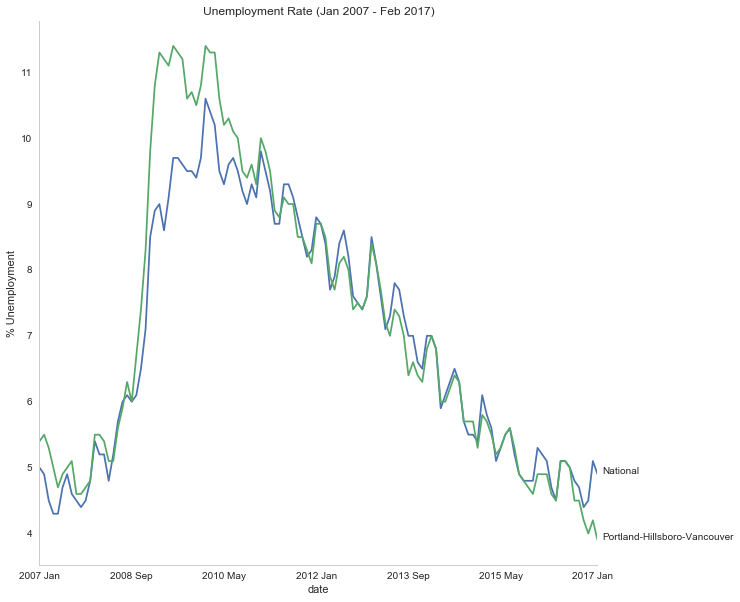## S&P 500

Now I'm going to compare the unemployment rate to the S&P 500 index for the same period. The S&P 500 data came from the Federal Reserve Bank of St. Louis. It contains the S&P 500 monthly index from May 2007 through February 2017.

### S and P Index

download_data(Paths.s_and_p, Urls.s_and_p)
s_and_p_index.describe()

pre = pandas.DataFrame({"DATE": ["2007-01-01", "2007-02-01", "2007-03-01"], "VALUE": [numpy.nan, numpy.nan, numpy.nan]})
s_and_p_index = pre.append(s_and_p_index)
s_and_p_index["date"] = portland.date.values
s_and_p_index = s_and_p_index.reset_index(drop=True)

s_and_p_index.tail()

s_and_p_nadir = s_and_p_index.VALUE.min()


So the stock-market hit bottom in December of 2008, six months before the Portland-Hillsboro-Vancouver unemployment rate reached its (first) high-point and ten months before the national unemployment rate hit its peak.

Next I'll see if plotting the S&P 500 Index vs Unemployment Rate data shows anything interesting.

figure = pyplot.figure(figsize=(10, 10))
axe = figure.gca()
# the S&P data is missing the first four months so slice
# the unemployment data
axe.plot(s_and_p_index.VALUE, national.unemployment_rate)
axe.plot(s_and_p_index.VALUE, portland.unemployment_rate)
axe.set_title("Unemployment Rate vs S&P 500")
axe.set_xlabel("S&P 500 Index")
axe.set_ylabel("% Unemployment")
last_x = s_and_p_index.VALUE.iloc[-1] + 100
axe.text(last_x, national.unemployment_rate.iloc[-1], "National")
axe.text(last_x, portland.unemployment_rate.iloc[-1], "Portland-Hillsboro-Vancouver")
seaborn.despine()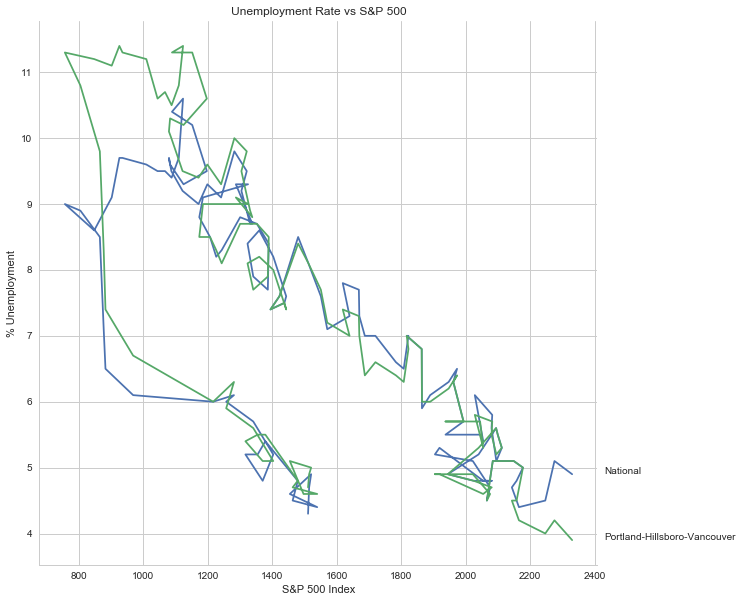It looks like as the S&P 500 goes down, the unemployment rate goes up, then, while the unemployment rate is at its peak, the S&P 500 starts to increase, even as the unemployment rate stays high, until around the time when it reached 1200, the unemployment rates began to go down as the stock market improved.

## Purchase Only House Price Index for the United States.

This data also came from the Federal Reserve Bank of St. Louis. It is based on more than six million repeat sales transactions on the same single-family properties. The original source of the data was the Federal Housing Finance Agency (but it only provides an xls file, not a csv, so I took it from the FED). From the FHFA:

The HPI is a broad measure of the movement of single-family house prices. The HPI is a weighted, repeat-sales index, meaning that it measures average price changes in repeat sales or refinancings on the same properties. This information is obtained by reviewing repeat mortgage transactions on single-family properties whose mortgages have been purchased or securitized by Fannie Mae or Freddie Mac since January 1975.

The HPI serves as a timely, accurate indicator of house price trends at various geographic levels. Because of the breadth of the sample, it provides more information than is available in other house price indexes. It also provides housing economists with an improved analytical tool that is useful for estimating changes in the rates of mortgage defaults, prepayments and housing affordability in specific geographic areas.

The HPI includes house ​price figures for the nine Census Bureau divisions, for the 50 states and the District of Columbia, and for Metropolitan Statistical Areas (MSAs) and Divisions.

download_data(Paths.house_price, Urls.house_price)
house_price_index.describe()

house_price_index.head()

house_price_index["price"] = house_price_index.HPIPONM226S
house_price_index["date"] = portland.date[1:].values

pre = pandas.DataFrame({"DATE": ["2007-01-01"], "HPIPONM226S": [numpy.nan], "price": [numpy.nan], "date": ["2007 Jan"]})
house_price_index = pre.append(house_price_index)
house_price_index = house_price_index.reset_index(drop=True)

house_price_index.tail()

housing_nadir = house_price_index.price.min()


The House Price Index hit its low point about two and a half years after the stock market hit its low point.

## The Final Plot

figure , axes = pyplot.subplots(3,
sharex=True)
(sp_axe, housing_axe, unemployment_axe) = axes
figure.set_size_inches(10, 10)

# plot the data
s_and_p_index.plot(x="date", y="VALUE", ax=sp_axe,
legend=False)
house_price_index.plot(x="date", y="price", ax=housing_axe,
legend=False)

national.plot(x="date", y="unemployment_rate", ax=unemployment_axe,
legend=False)
portland.plot(x="date", y="unemployment_rate", ax=unemployment_axe,
legend=False)

# plot the peaks/low-points as vertical lines
peak_color = "darkorange"
# portland-unemployment peaks
for peak in unemployment_peaks:
for axe in axes:
axe.axvline(peak, color=peak_color)

(national_peak, "grey"))

for point, color in points:
for axe in axes:
axe.axvline(point, color=color)

# level at the start of the recession (it was the same for both Portland and the U.S.)
unemployment_axe.axhline(national.unemployment_rate.iloc[recession_start], alpha=0.25)
housing_axe.axhline(
house_price_index.price.iloc[
numpy.where(house_price_index.date=="2007 Dec")], alpha=0.25)
sp_axe.axhline(
s_and_p_index.VALUE.iloc[
numpy.where(s_and_p_index.date=="2007 Dec")], alpha=0.25)

unemployment_axe.set_ylabel("% Unemployment")
unemployment_axe.set_xlabel("")

housing_axe.set_ylabel("Sale Price (\$1,000)")
sp_axe.set_ylabel("S&P 500 Index")

figure.suptitle("Unemployment Rate April 2007 To February 2017 with S&P 500 Index and House Price Index",
weight="bold")

# label the data lines
last = portland.date.count()
unemployment_axe.text(last, national.unemployment_rate.iloc[-1], "National")
unemployment_axe.text(last, portland.unemployment_rate.iloc[-1], "Portland-Hillsboro-Vancouver")
sp_axe.text(last, s_and_p_index.VALUE.iloc[-1], "S&P 500")
housing_axe.text(last, house_price_index.price.iloc[-1], "House Price Index")

# color in the recession
sp_axe.axvspan(recession_start, recession_end, alpha=0.25, facecolor='royalblue')
housing_axe.axvspan(recession_start, recession_end, alpha=0.25, facecolor='royalblue')
unemployment_axe.axvspan(recession_start, recession_end, alpha=0.25, facecolor='royalblue')

# label the vertical lines
sp_axe.text(s_and_p_nadir, s_and_p_index.VALUE.max() + 450, "S&P Low", rotation=45)
sp_axe.text(unemployment_peaks, s_and_p_index.VALUE.max() + 575,  "Portland High", rotation=45)
sp_axe.text(housing_nadir, s_and_p_index.VALUE.max() + 550, "Housing Low", rotation=45)
sp_axe.text(36, s_and_p_index.VALUE.max() + 450, "U.S. High", rotation=45)
seaborn.despine()

# the coursera sight gives you the option to add a caption via the GUI
# figure.text(.1,.000001, """
#    Monthly Unadjusted Unemployment Rates for the Portland-Hillsboro-Vancouver area and the entire United States of America compared with the S&P 500 Index and
#    House Price Index for the same period. The blue highlighted area is a period of economic contraction (December 2007 through June 2009) defined by the National
#    Bureau of Economic Research. The vertical lines represent (red) the low-point for the S&P 500, (orange) the first peak of the Portland-Hillsboro-Vancouver area
#    unemployment, (gray) the peak of U.S. unemployment (overlaps second Portland-area value matching its first peak), and (green) the low-point for the house-price index.
#    The horizontal lines are the values for the metrics at the start of the recession.""")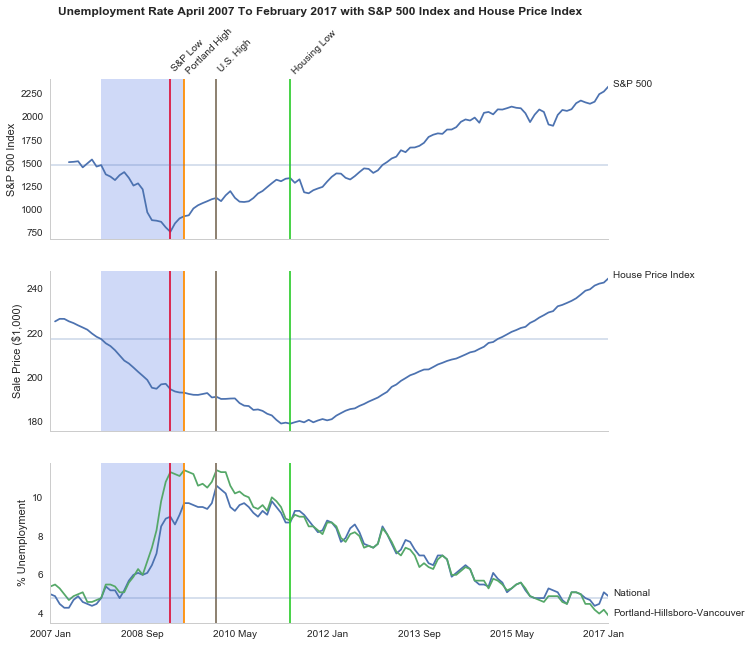line = pygal.Line()
line.x_labels = s_and_p_index.date
line.render_to_file("unemployment_portland_vs_us_2004_2017.svg")
# plot the data
# the coursera sight gives you the option to add a caption via the GUI
# figure.text(.1,.000001, """
#    Monthly Unadjusted Unemployment Rates for the Portland-Hillsboro-Vancouver area and the entire United States of America compared with the S&P 500 Index and
#    House Price Index for the same period. The blue highlighted area is a period of economic contraction (December 2007 through June 2009) defined by the National
#    Bureau of Economic Research. The vertical lines represent (red) the low-point for the S&P 500, (orange) the first peak of the Portland-Hillsboro-Vancouver area
#    unemployment, (gray) the peak of U.S. unemployment (overlaps second Portland-area value matching its first peak), and (green) the low-point for the house-price index.
#    The horizontal lines are the values for the metrics at the start of the recession.""")


The visualization created was meant to show how Portland, Oregon, United States' unemployment rate related to the national unemployment rate, the stock market, and housing prices. The seasonally unadjusted employment rates for the Portland-Vancouver-Hillsboro area were retrieved from the Bureau of Labor Statistics' web-site, along with the unadjusted unemployment rates for the nation as a whole for the months from January 2017 through February 2017. Hillsboro is an incorporated part of metropolitan Portland and Vancouver is just North of Portland so many of its residents commute to Portland to work, and vice-versa. The monthly S&P 500 Index from May 2007 through February 2017 along with the Purchase Only Price Index from February 2007 through February 2017 were retrieved from the St. Louis Federal Reserve website. The S&P 500 index is the market capitalization of 500 large companies listed on the New York Stock Exchange or NASDAQ. The Purchase Only House Price Index is the average price change in repeat sales or refinancing of the same houses and is maintained by Federal Housing Finance Agency. The beginning and ending of the recession within this time period was taken from the National Bureau of Economic Research (https://www.nber.org/cycles.html).

The visualization shows that during the recession, beginning in roughly September 2008, Portland's unemployment rate rose faster than the nation as a whole did, but by roughly May 2011 (coinciding with the lowest valuation for the House Price Index) it had dropped slightly lower than the national rate and has stayed in step with it, although it has thus far not followed the uptick in the national rate that began in November of 2016. Additionally the visualization shows the relative timing of the changes in the three metrics. In the year leading up to the recession, unemployment was relatively flat (ignoring the seasonal changes) and the S&P also began relatively flat but then began a downward trend later in the year, the House Price Index, on the other hand, spent most of it starting what would become a four-year decline (since this was during the sub-prime mortgage crisis, this is perhaps not so surprising). The S&P 500 hit its low point during the recession, as might be expected, but the peaks for the unemployment rates occurred when the recession was already over. Also, while the S&P 500 recovered relatively quickly, the unemployment rates for both Portland and the United States as a whole did not reach the level that they were at when the recession began until October 2015.

Truthfulness:

To provide a baseline of trustworthiness I used only government sources (although, of course, some might see that as a negative).

Beauty:

The internal grid was left out and in its place only vertical and horizontal lines for key values were highlighted (the vertical line represent the worst points for each metric, the horizontal lines the values that the metrics held when the recession began - so the point at which the horizontal line intersects the line after the recession is its recovery point) in an attempt to increase the data-ink ratio.

Functionality:

The data was plotted with a shared x-axis and three separate y-axes so that the states of each could be compared at the same point in time without distorting the plots due to the differing scales for each metric. I didn't include 0 on the y-axes, but the point was to observe inflection points and trends rather than measure exact values so I felt that this was unnecessary (it added a lot of whitespace without actually changing the shapes). As mentioned in the previous section, key points in the data were highlighted (including the time of the recession) so that the viewer could have some additional background information with regard to what was happening, and not just wonder what the strange spike in unemployment was about (or needing to know all the dates ahead of time).

Insightfulness:

By comparing the Portland unemployment rates to the national rates it hopefully revealed the story of how Portland did with regards to the rest of the country - initially doing worse than the nation, then catching up, and currently doing a little better. Additionally, by adding the context of the recession, as well as the performance of the S&P 500 index and the House Price Index during the same period, I hoped to show how unemployment (at least in this time period) moved in relation to other parts of the economy.

# Plotting With Uncertainty (Part III)

This is an implementation of the harder option for Assignment 3 of coursera's Applied Plotting, Charting & Data Representation in Python.

## Description¶

A challenge that users face is that, for a given y-axis value (e.g. 42,000), it is difficult to know which x-axis values are most likely to be representative, because the confidence levels overlap and their distributions are different (the lengths of the confidence interval bars are unequal). One of the solutions the authors propose for this problem (Figure 2c) is to allow users to indicate the y-axis value of interest (e.g. 42,000) and then draw a horizontal line and color bars based on this value. So bars might be colored red if they are definitely above this value (given the confidence interval), blue if they are definitely below this value, or white if they contain this value.

Even Harder option: Add interactivity to the above, which allows the user to click on the y axis to set the value of interest. The bar colors should change with respect to what value the user has selected.

## Imports¶

All the imports were created by third-parties (taken from pypi).

In :
import matplotlib.pyplot as pyplot
import numpy
import pandas
import scipy.stats as stats
import seaborn


## Some Plotting Setup¶

In :
%matplotlib notebook
style = seaborn.axes_style("whitegrid")
style["axes.grid"] = False
seaborn.set_style("whitegrid", style)


## The Data¶

There data set will be four normally-distributed, randomly generated data sets each representing a simulated data set for a given year.

### numpy.random.normal¶

This is from the numpy.random.normal doc-string:

normal(loc=0.0, scale=1.0, size=None)

Draw random samples from a normal (Gaussian) distribution.

The probability density function of the normal distribution, first derived by De Moivre and 200 years later by both Gauss and Laplace independently _, is often called the bell curve because of its characteristic shape (see the example below).

The normal distributions occurs often in nature. For example, it describes the commonly occurring distribution of samples influenced by a large number of tiny, random disturbances, each with its own unique distribution.

### Parameters¶

#### loc : float or array_like of floats¶

Mean ("centre") of the distribution.

#### scale : float or array_like of floats¶

Standard deviation (spread or "width") of the distribution.

#### size : int or tuple of ints, optional¶

Output shape. If the given shape is, e.g., (m, n, k), then m * n * k samples are drawn. If size is None (default), a single value is returned if loc and scale are both scalars. Otherwise, np.broadcast(loc, scale).size samples are drawn.

In :
numpy.random.seed(12345)

data = pandas.DataFrame([numpy.random.normal(33500,150000,3650),
numpy.random.normal(41000,90000,3650),
numpy.random.normal(41000,120000,3650),
numpy.random.normal(48000,55000,3650)],
index=[1992,1993,1994,1995])

In :
data.T.describe()

Out:
1992 1993 1994 1995
count 3650.000000 3650.000000 3650.000000 3650.000000
mean 34484.080607 39975.673587 37565.689950 47798.504333
std 150473.176164 88558.520583 120317.078777 54828.074297
min -528303.381600 -287127.421315 -382709.382654 -138894.685422
25% -67555.298773 -21665.471992 -45516.912051 11680.007981
50% 31756.198287 41001.778992 39197.241173 49103.396625
75% 135081.379021 99766.920131 121367.183244 84271.976610
max 622629.206119 358327.854561 423792.855746 262363.983464

Comparing the sample to the values fed to the normal function it appears that even with 3,650 values, it's still not exactly what we asked for.

In :
data.T.plot.kde()
seaborn.despine()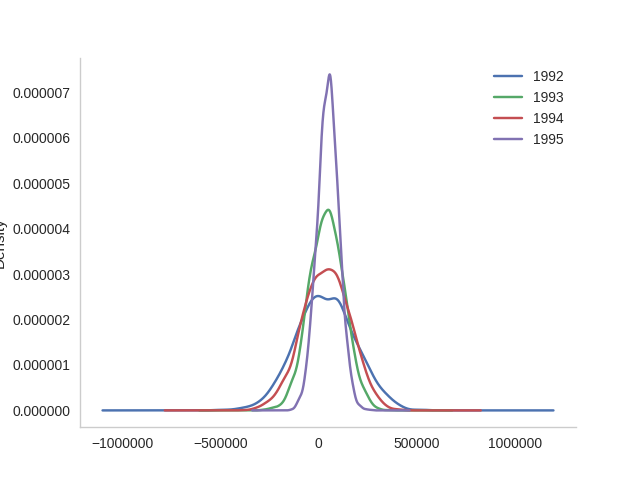1992, the plot with the largest spread looks kind of lumpy. Their means look surprisingly close, but that's probably because the large standaard deviation distorts the scale.

In :
data.T.plot.box()
seaborn.despine()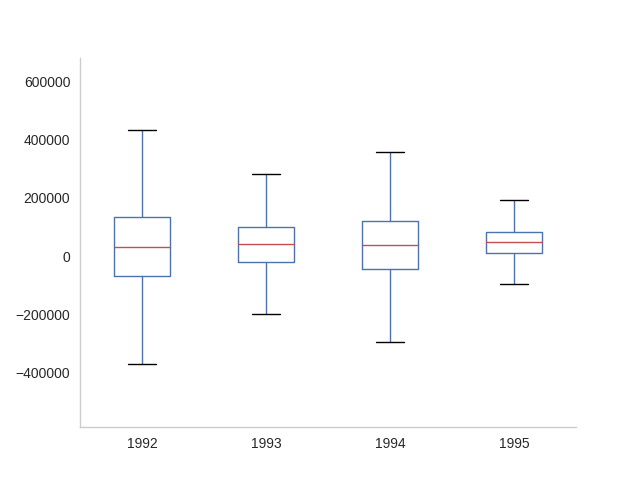The box-plot shows once again that there centers are relatively close. But 1992 and 1994 have considerably more spread than 1993 and especially more than 1995.

## Interval Check¶

This is the class that implements the plotting. It colors the bar-plots based on whether the value given is within a bar's confidence interval (white), below the confidence interval (blue) or above the confidence interval (red). It's set up to work with the easiest case so the color_bars method has to be overridden to make it work for this case.

In :
class IntervalCheck(object):
"""colors plot based on whether a value is in range
Args:
data (DataFrame): frame with data of interest as columns
confidence_interval (float): probability we want to exceed
"""
def __init__(self, data, confidence_interval=0.95, title="Confidence Intervals"):
self.data = data
self.confidence_interval = confidence_interval
self.title = title
self._intervals = None
self._lows = None
self._highs = None
self._errors = None
self._means = None
self._errors = None
self._figure = None
self._axes = None
self._bars = None
self.horizontal_line = None
self.line_label = None
return

@property
def figure(self):
if self._figure is None:
"""A pyplot figure"""
self._figure = pyplot.figure()
return self._figure

@property
def axes(self):
if self._axes is None:
"""the current axes for self.figure"""
self._axes = self.figure.gca()
self._axes.set_title(self.title)
return self._axes

@property
def bars(self):
"""the bar-plot-objects"""
if self._bars is None:
self._bars = self.axes.bar(self.data.columns, self.means,
yerr=self.errors)
return self._bars

@property
def intervals(self):
"""list of high and low interval tuples"""
if self._intervals is None:
data = (self.data[column] for column in self.data)
self._intervals = [stats.norm.interval(alpha=self.confidence_interval,
loc=datum.mean(),
scale=datum.sem())
for datum in data]
return self._intervals

@property
def lows(self):
"""the low-ends for the confidence intervals
Returns:
numpy.array of low-end confidence interval values
"""
if self._lows is None:
self._lows = numpy.array([low for low, high in self.intervals])
return self._lows

@property
def highs(self):
"""high-ends for the confidence intervals
Returns:
numpy.array of high-end values for confidence intervals
"""
if self._highs is None:
self._highs = numpy.array([high for low, high in self.intervals])
return self._highs

@property
def means(self):
"""the means of the data-arrays"""
if self._means is None:
self._means = self.data.mean()
return self._means

@property
def errors(self):
"""The size of the errors, rather than the ci values"""
if self._errors is None:
self._errors = self.highs - self.means
return self._errors

def print_intervals(self):
"""print org-mode formatted table of the confidence intervals"""
intervals = pandas.DataFrame({column: self.intervals[index]
for index, column in enumerate(self.data.columns)},
index="low high".split())
try:
except NameError:
# not supported
pass
return

def draw_value(self, value):
"""draws the horizontal line and value"""
if self.horizontal_line:
self.horizontal_line.set_ydata(value)
self.line_label.set_y(value)
self.line_label.set_text("{0:.2f}".format(value))
else:
self.horizontal_line = pyplot.axhline(value,
axes=self.axes,
color="darkorange")
self.line_label = pyplot.text(self.data.columns,
value,
"{0:.2f}".format(value),
axes=self.axes,
bbox={"facecolor": "white", "boxstyle": "round"})
return

def setup_bars(self, value):
"""sets up the horizontal line, value and bars
Args:
value (float): value to compare to distributions
"""
x_labels = [str(index) for index in self.data.columns]
for bar in self.bars:
bar.set_edgecolor("royalblue")
pyplot.xticks(self.data.columns, x_labels)
self.draw_value(value)
return

def color_bars(self, value):
"""colors the bars based on the value
this is the easiest case
Args:
value (float): value to compare to the distribution
"""
for index, bar in enumerate(self.bars):
if value < self.lows[index]:
bar.set_color('crimson')
elif self.lows[index] <= value <= self.highs[index]:
bar.set_color('w')
bar.set_edgecolor("royalblue")
else:
bar.set_color("royalblue")
return

def __call__(self, value):
"""plots the data and value
* blue bar if value above c.i.
* white bar if value in c.i.
* red bar if value is below c.i.

Args:
value (float): what to compare to the data
"""
self.setup_bars(value)
self.color_bars(value)
return


## Harder¶

This is the class that implements the harder coloring scheme were a gradient is used instead of just three colors.

In :
class Harder(IntervalCheck):
"""implements the harder problem
"""
def __init__(self, *args, **kwargs):
super(Harder, self).__init__(*args, **kwargs)
self._colors = None
self._proportions = None
return

@property
def colors(self):
"""array of rgb color triples"""
if self._colors is None:
# could have been done with straight fractions
# but I find it easier to think in terms of
# 0..255
base = list(range(0, 255, 51))
full =  * 6
blue = numpy.array(base + full)
blue = blue/255
base.reverse()
red = numpy.array(full + base)
red = red/255
tail = base[:]
base.reverse()
green = numpy.array(base +  + tail)/255
self._colors = numpy.array([red, green, blue]).T
return self._colors

@property
def proportions(self):
"""array of upper limits for the value to find the matching color
"""
if self._proportions is None:
self._proportions = numpy.linspace(0.09, 1, 10)
return self._proportions

def color_bars(self, value):
"""colors the bars based on the value
this is the harder case
Args:
value (float): value to compare to the distribution
"""
mapped_values = [(value - low)/(high - low)
for low, high in self.intervals]
for index, mapped_value in enumerate(mapped_values):
if mapped_value < 0:
self.bars[index].set_color(self.colors)
continue
if mapped_value >= 1:
self.bars[index].set_color(self.colors[-1])
continue
for p_index, proportion in enumerate(self.proportions):
if mapped_value <= proportion:
color = self.colors[p_index]
self.bars[index].set_color(color)
self.bars[index].set_edgecolor("royalblue")
break
return


## Even Harder¶

This is the class that adds interactivity to the Harder case.

In :
class EvenHarder(Harder):
"""the interactive version of Harder"""
@property
def figure(self):
"""pyplot figure
As a side-effect registers on_click with the canvas
"""
if self._figure is None:
self._figure = pyplot.figure()
self._figure.canvas.mpl_connect("button_press_event",
self.on_click)
return self._figure

def on_click(self, event):
"""event-handler to update the plot"""
if event.ydata:
self.draw_value(event.ydata)
self.color_bars(event.ydata)
return
def __call__(self, value=0):
"""add a default value since this is interactive"""
super(EvenHarder, self).__call__(value)
return


## Examples¶

First, I'll take a look at the values for the confidence intervals so that I can find values to plot. Here are the confidence intervals for the data I created.

In :
plotter = EvenHarder(data=data.T)
plotter.print_intervals()


Here's a value that is below all the confidence intervals.

In :
value = 42000
plotter(value)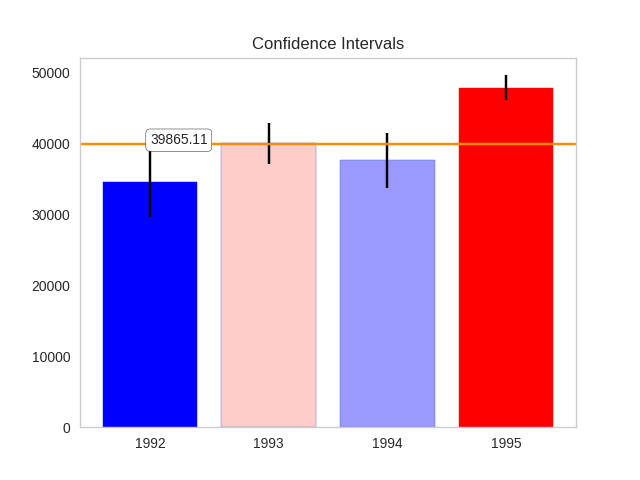# Plotting With Uncertainty (Part II)

This is an implementation of the harder option for Assignment 3 of coursera's Applied Plotting, Charting & Data Representation in Python.

## Description

A challenge that users face is that, for a given y-axis value (e.g. 42,000), it is difficult to know which x-axis values are most likely to be representative, because the confidence levels overlap and their distributions are different (the lengths of the confidence interval bars are unequal). One of the solutions the authors propose for this problem (Figure 2c) is to allow users to indicate the y-axis value of interest (e.g. 42,000) and then draw a horizontal line and color bars based on this value. So bars might be colored red if they are definitely above this value (given the confidence interval), blue if they are definitely below this value, or white if they contain this value.

Harder option: Implement the bar coloring as described in the paper, where the color of the bar is actually based on the amount of data covered (e.g. a gradient ranging from dark blue for the distribution being certainly below this y-axis, to white if the value is certainly contained, to dark red if the value is certainly not contained as the distribution is above the axis).

## Imports

All the imports were created by third-parties (taken from pypi).

from tabulate import tabulate
import matplotlib.pyplot as pyplot
import numpy
import pandas
import scipy.stats as stats
import seaborn


### Some Plotting Setup

%matplotlib inline
style = seaborn.axes_style("whitegrid")
style["axes.grid"] = False
seaborn.set_style("whitegrid", style)


## The Data

There data set will be four normally-distributed, randomly generated data sets each representing a simulated data set for a given year.

### numpy.random.normal

This is from the numpy.random.normal doc-string:

normal(loc=0.0, scale=1.0, size=None)

Draw random samples from a normal (Gaussian) distribution.

The probability density function of the normal distribution, first derived by De Moivre and 200 years later by both Gauss and Laplace independently 1_, is often called the bell curve because of its characteristic shape (see the example below).

The normal distributions occurs often in nature. For example, it describes the commonly occurring distribution of samples influenced by a large number of tiny, random disturbances, each with its own unique distribution.

### Parameters

#### loc : float or arraylike of floats

Mean ("centre") of the distribution.

#### scale : float or arraylike of floats

Standard deviation (spread or "width") of the distribution.

#### size : int or tuple of ints, optional

Output shape. If the given shape is, e.g., (m, n, k), then m * n * k samples are drawn. If size is None (default), a single value is returned if loc and scale are both scalars. Otherwise, np.broadcast(loc, scale).size samples are drawn.

numpy.random.seed(12345)

data = pandas.DataFrame([numpy.random.normal(33500,150000,3650),
numpy.random.normal(41000,90000,3650),
numpy.random.normal(41000,120000,3650),
numpy.random.normal(48000,55000,3650)],
index=[1992,1993,1994,1995])

description = data.T.describe()


Comparing the sample to the values fed to the normal function it appears that even with 3,650 values, it's still not exactly what we asked for.

data.T.plot.kde()
seaborn.despine()


1992, the plot with the largest spread looks kind of lumpy. Their means look surprisingly close, but that's probably because the large standaard deviation distorts the scale.

data.T.plot.box()
seaborn.despine()


The box-plot shows once again that there centers are relatively close. But 1992 and 1994 have considerably more spread than 1993 and especially more than 1995.

## Interval Check

This is the class that implements the plotting. It colors the bar-plots based on whether the value given is within a bar's confidence interval (white), below the confidence interval (blue) or above the confidence interval (red). It's set up to work with the easiest case so the color_bars method has to be overridden to make it work for this case.

class IntervalCheck(object):
"""colors plot based on whether a value is in range
Args:
data (DataFrame): frame with data of interest as columns
confidence_interval (float): probability we want to exceed
"""
def __init__(self, data, confidence_interval=0.95):
self.data = data
self.confidence_interval = confidence_interval
self._intervals = None
self._lows = None
self._highs = None
self._errors = None
self._means = None
self._errors = None
return

@property
def intervals(self):
"""list of high and low interval tuples"""
if self._intervals is None:
data = (self.data[column] for column in self.data)
self._intervals = [stats.norm.interval(alpha=self.confidence_interval,
loc=datum.mean(),
scale=datum.sem())
for datum in data]
return self._intervals

@property
def lows(self):
"""the low-ends for the confidence intervals
Returns:
numpy.array of low-end confidence interval values
"""
if self._lows is None:
self._lows = numpy.array([low for low, high in self.intervals])
return self._lows

@property
def highs(self):
"""high-ends for the confidence intervals
Returns:
numpy.array of high-end values for confidence intervals
"""
if self._highs is None:
self._highs = numpy.array([high for low, high in self.intervals])
return self._highs

@property
def means(self):
"""the means of the data-arrays"""
if self._means is None:
self._means = self.data.mean()
return self._means

@property
def errors(self):
"""The size of the errors, rather than the ci values"""
if self._errors is None:
self._errors = self.highs - self.means
return self._errors

def print_intervals(self):
"""print org-mode formatted table of the confidence intervals"""
intervals = pandas.DataFrame({column: self.intervals[index]
for index, column in enumerate(self.data.columns)},
index="low high".split())
try:
except ImportError:
# not supported
pass
return

def setup_bars(self, value):
"""sets up the horizontal line, value and bars
Args:
value (float): value to compare to distributions
Returns:
bars (list): collection of bar-plot objects for the data
"""
figure = pyplot.figure()
axe = figure.gca()

x_labels = [str(index) for index in self.data.columns]
bars = axe.bar(self.data.columns, self.means, yerr=self.errors)
for bar in bars:
bar.set_edgecolor("royalblue")
pyplot.xticks(self.data.columns, x_labels)
pyplot.axhline(value, color='darkorange')
pyplot.text(self.data.columns, value, str(value),
bbox={"facecolor": "white", "boxstyle": "round"})
return bars

def color_bars(self, value, bars):
"""colors the bars based on the value
this is the easiest case
Args:
value (float): value to compare to the distribution
bars (list): list of bar-plot objects created from data
"""
for index, bar in enumerate(bars):
if value < self.lows[index]:
bar.set_color('crimson')
elif self.lows[index] <= value <= self.highs[index]:
bar.set_color('w')
bar.set_edgecolor("royalblue")
else:
bar.set_color("royalblue")
return

def __call__(self, value):
"""plots the data and value
* blue bar if value above c.i.
* white bar if value in c.i.
* red bar if value is below c.i.

Args:
value (float): what to compare to the data
"""
bars = self.setup_bars(value)
self.color_bars(value, bars)
return


## Harder

This is the class that implements the harder coloring scheme were a gradient is used instead of just three colors.

class Harder(IntervalCheck):
"""implements the harder problem
"""
def __init__(self, *args, **kwargs):
super(Harder, self).__init__(*args, **kwargs)
self._colors = None
self._proportions = None
return

@property
def colors(self):
"""array of rgb color triples"""
if self._colors is None:
# could have been done with straight fractions
# but I find it easier to think in terms of
# 0..255
base = list(range(0, 255, 51))
full =  * 6
blue = numpy.array(base + full)
blue = blue/255
base.reverse()
red = numpy.array(full + base)
red = red/255
tail = base[:]
base.reverse()
green = numpy.array(base +  + tail)/255
self._colors = numpy.array([red, green, blue]).T
return self._colors

@property
def proportions(self):
"""array of upper limits for the value to find the matching color
"""
if self._proportions is None:
self._proportions = numpy.linspace(0.09, 1, 10)
return self._proportions

def color_bars(self, value, bars):
"""colors the bars based on the value
this is the harder case
Args:
value (float): value to compare to the distribution
bars (list): list of bar-plot objects created from data
"""
mapped_values = [(value - low)/(high - low)
for low, high in self.intervals]
for index, mapped_value in enumerate(mapped_values):
for p_index, proportion in enumerate(self.proportions):
if mapped_value < proportion:
color = self.colors[p_index]
bars[index].set_color(color)
bars[index].set_edgecolor("royalblue")
break
return


## Examples

First, I'll take a look at the values for the confidence intervals so that I can find values to plot. Here are the confidence intervals for the data I created.

plotter = Harder(data=data.T)
plotter.print_intervals()


Here's a value that is below all the confidence intervals. as you can see, all the bars are red.

value = 29000
# value = 33000
# value = 39974
# value = 42000
# value = 48000
# value = 49600
plotter(value)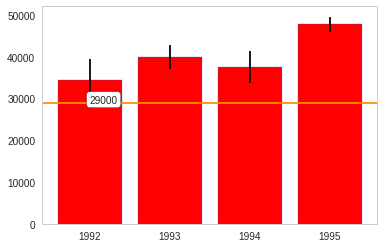For the next value 1992 is light blue, so the value is slightly above the mean, while 1994 is light red, so the value is within the confidence interval but below the mean.

value = 36000
plotter(value)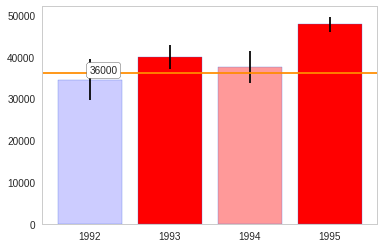This next value is at or close to 1993's mean and slightly above 1994's mean.

value = 39974
# value = 42000
# value = 48000
# value = 49600
plotter(value)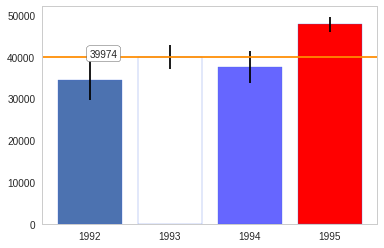This next value is within 1993's confidence interval, but it is light blue so it's above the mean.

value = 42000
plotter(value)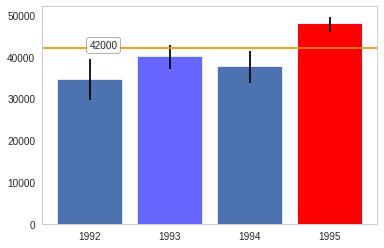The next value is within 1995's confidence interval but the darker blue indicates that it is nearly above the interval.

value = 49500
plotter(value)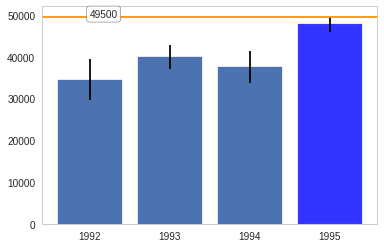And finally, a value that's above all the confidence intervals.

value = 50000
plotter(value)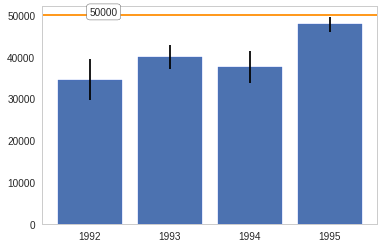1

# Plotting With Uncertainty (Part I)

This is an implementation of the easiest option for Assignment 3 of coursera's Applied Plotting, Charting & Data Representation in Python.

## Description

In this paper the authors describe the challenges users face when trying to make judgements about probabilistic data generated through samples. As an example, they look at a bar chart of four years of data (replicated below in Figure 1). Each year has a y-axis value, which is derived from a sample of a larger dataset. For instance, the first value might be the number votes in a given district or riding for 1992, with the average being around 33,000. On top of this is plotted the confidence interval – the range of the number of votes which encapsulates 95% of the data (see the boxplot lectures for more information, and the yerr parameter of barcharts).

A challenge that users face is that, for a given y-axis value (e.g. 42,000), it is difficult to know which x-axis values are most likely to be representative, because the confidence levels overlap and their distributions are different (the lengths of the confidence interval bars are unequal). One of the solutions the authors propose for this problem (Figure 2c) is to allow users to indicate the y-axis value of interest (e.g. 42,000) and then draw a horizontal line and color bars based on this value. So bars might be colored red if they are definitely above this value (given the confidence interval), blue if they are definitely below this value, or white if they contain this value.

Easiest option: Implement the bar coloring as described above - a color scale with only three colors, (e.g. blue, white, and red). Assume the user provides the y axis value of interest as a parameter or variable.

## Imports

All the imports were created by third-parties (taken from pypi).

from tabulate import tabulate
import matplotlib.pyplot as pyplot
import numpy
import pandas
import scipy.stats as stats
import seaborn


### Some Plotting Setup

%matplotlib inline
style = seaborn.axes_style("whitegrid")
style["axes.grid"] = False
seaborn.set_style("whitegrid", style)


## The Data

The data set will be four normally-distributed, randomly generated, data sets each representing a simulated data set for a given year.

### numpy.random.normal

This is from the numpy.random.normal doc-string:

normal(loc=0.0, scale=1.0, size=None)

Draw random samples from a normal (Gaussian) distribution.

The probability density function of the normal distribution, first derived by De Moivre and 200 years later by both Gauss and Laplace independently1, is often called the bell curve because of its characteristic shape (see the example below).

The normal distributions occurs often in nature. For example, it describes the commonly occurring distribution of samples influenced by a large number of tiny, random disturbances, each with its own unique distribution2.

#### Parameters

• loc : float or array-like of floats

Mean ("centre") of the distribution.

• scale : float or array-like of floats

Standard deviation (spread or "width") of the distribution.

• size : int or tuple of ints, optional

Output shape. If the given shape is, e.g., (m, n, k), then m * n * k samples are drawn. If size is None (default), a single value is returned if loc and scale are both scalars. Otherwise, np.broadcast(loc, scale).size samples are drawn.

### Creating the data

numpy.random.seed(12345)

data = pandas.DataFrame([numpy.random.normal(33500,150000,3650),
numpy.random.normal(41000,90000,3650),
numpy.random.normal(41000,120000,3650),
numpy.random.normal(48000,55000,3650)],
index=[1992,1993,1994,1995])

description = data.T.describe()

1992 1993 1994 1995
count 3650 3650 3650 3650
mean 34484.1 39975.7 37565.7 47798.5
std 150473 88558.5 120317 54828.1
min -528303 -287127 -382709 -138895
25% -67555.3 -21665.5 -45516.9 11680
50% 31756.2 41001.8 39197.2 49103.4
75% 135081 99766.9 121367 84272
max 622629 358328 423793 262364

Comparing the sample to the values fed to the normal function it appears that even with 3,650 values, it's still not exactly what we asked for.

data.T.plot.kde()
seaborn.despine()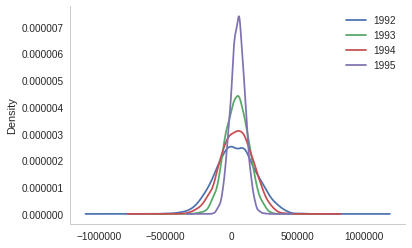1992, the plot with the largest spread looks kind of lumpy. Their means look surprisingly close, but that's probably because the large standaard deviation distorts the scale.

data.T.plot.box()
seaborn.despine()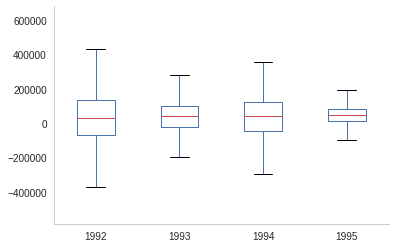The box-plot shows once again that the centers are relatively close. But 1992 and 1994 have considerably more spread than 1993 and especially more than 1995.

## Interval Check

This is the class that implements the plotting. It colors the bar-plots based on whether the value given is within a bar's confidence interval (white), below the confidence interval (blue) or above the confidence interval (red).

class IntervalCheck(object):
"""colors plot based on whether a value is in range
Args:
data (DataFrame): frame with data of interest as columns
confidence_interval (float): probability we want to exceed
"""
def __init__(self, data, confidence_interval=0.95):
self.data = data
self.confidence_interval = confidence_interval
self._intervals = None
self._lows = None
self._highs = None
self._errors = None
self._means = None
self._errors = None
return

@property
def intervals(self):
"""list of high and low interval tuples"""
if self._intervals is None:
data = (self.data[column] for column in self.data)
self._intervals = [stats.norm.interval(alpha=self.confidence_interval,
loc=datum.mean(),
scale=datum.sem())
for datum in data]
return self._intervals

@property
def lows(self):
"""the low-ends for the confidence intervals
Returns:
numpy.array of low-end confidence interval values
"""
if self._lows is None:
self._lows = numpy.array([low for low, high in self.intervals])
return self._lows

@property
def highs(self):
"""high-ends for the confidence intervals
Returns:
numpy.array of high-end values for confidence intervals
"""
if self._highs is None:
self._highs = numpy.array([high for low, high in self.intervals])
return self._highs

@property
def means(self):
"""the means of the data-arrays"""
if self._means is None:
self._means = self.data.mean()
return self._means

@property
def errors(self):
"""The size of the errors, rather than the ci values"""
if self._errors is None:
self._errors = self.highs - self.means
return self._errors

def print_intervals(self):
"""print org-mode formatted table of the confidence intervals"""
intervals = pandas.DataFrame({column: self.intervals[index]
for index, column in enumerate(self.data.columns)},
index="low high".split())
try:
except ImportError:
# not supported
pass
return

def __call__(self, value):
"""plots the data and value
* blue bar if value above c.i.
* white bar if value in c.i.
* red bar if value is below c.i.

Args:
value (float): what to compare to the data
"""
figure = pyplot.figure()
axe = figure.gca()

x_labels = [str(index) for index in self.data.columns]
bars = axe.bar(self.data.columns, self.means, yerr=self.errors)
pyplot.xticks(self.data.columns, x_labels)
pyplot.axhline(value, color='darkorange')
pyplot.text(self.data.columns, value, str(value),
bbox={"facecolor": "white", "boxstyle": "round"})
for index, bar in enumerate(bars):
if value < self.lows[index]:
bar.set_color('crimson')
elif self.lows[index] <= value <= self.highs[index]:
bar.set_color('w')
bar.set_edgecolor("royalblue")
else:
bar.set_color("royalblue")
return


## Examples

First, I'll take a look at the values for the confidence intervals so that I can find values to plot. Here are the confidence intervals for the data I created.

plotter = IntervalCheck(data=data.T)
plotter.print_intervals()

1992 1993 1994 1995
low 29602.5 37102.7 33662.4 46019.8
high 39365.7 42848.6 41469 49577.2

Here's a value that is below all the confidence intervals.

value = 29000
plotter(value)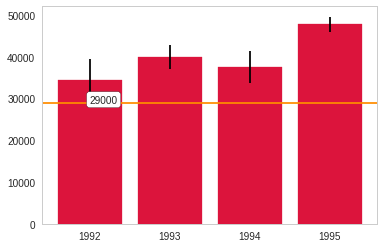Here's a value that is within 1992's confidence interval but below the other years.

value = 33000
plotter(value)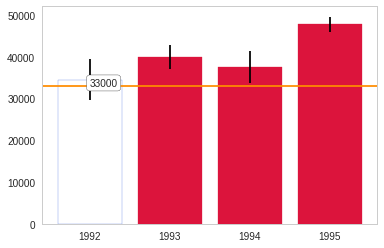Here's a value within 1993's and 1994's confidence intervals, but above 1992's and below 1995's confidence intervals.

value = 39974
plotter(value)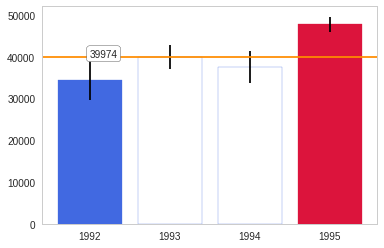Here's a value that is within 1993's confidence interval only.

value = 42000
plotter(value)Here's a value withing only 1995's confidence interval.

value = 49500
plotter(value)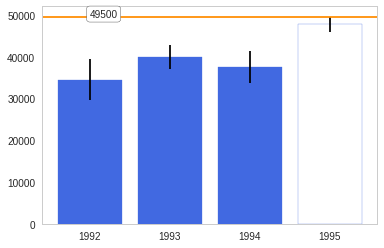And finally, a value that's above all the confidence intervals.

value = 50000
plotter(value)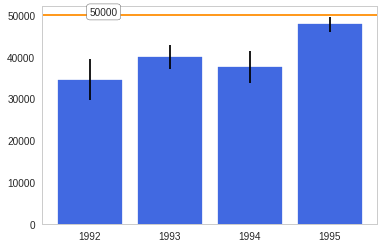## Footnotes:

1

Wikipedia, "Normal distribution", http://en.wikipedia.org/wiki/Normal_distribution

2

P. R. Peebles Jr., "Central Limit Theorem" in "Probability, Random Variables and Random Signal Principles", 4th ed., 2001, pp. 51, 51, 125.

# Assignment 2 - Pandas Introduction (Olympic Medals)

This is the notebook for assignment 2 of the Coursera Python Data Analysis course. This was converted from a jupyter notebook that you can download it as part of the course downloads zip file. The submissions work by uploading a ipynb file so there's a bit of cutting and pasting needed to get the code from here to there. Also I didn't use their variable names so the final outcome needs some aliasing for the grader to pass it.

## Part 1

The following code loads the olympics dataset (olympics.csv), which was derived from the Wikipedia entry on All Time Olympic Games Medals, and does some basic data cleaning.

The columns are organized as

• # of Summer games,
• Summer medals
• # of Winter games
• Winter medals
• total # number of games
• total # of medals

### Imports

# third party
import numpy
import pandas
from tabulate import tabulate


#### A quick look at the data-source

The data is stored in a comma-separated file named olympics.csv.

head olympics.csv

0,1,2,3,4,5,6,7,8,9,10,11,12,13,14,15
,№ Summer,01 !,02 !,03 !,Total,№ Winter,01 !,02 !,03 !,Total,№ Games,01 !,02 !,03 !,Combined total
Afghanistan (AFG),13,0,0,2,2,0,0,0,0,0,13,0,0,2,2
Algeria (ALG),12,5,2,8,15,3,0,0,0,0,15,5,2,8,15
Argentina (ARG),23,18,24,28,70,18,0,0,0,0,41,18,24,28,70
Armenia (ARM),5,1,2,9,12,6,0,0,0,0,11,1,2,9,12
Australasia (ANZ) [ANZ],2,3,4,5,12,0,0,0,0,0,2,3,4,5,12
Australia (AUS) [AUS] [Z],25,139,152,177,468,18,5,3,4,12,43,144,155,181,480
Austria (AUT),26,18,33,35,86,22,59,78,81,218,48,77,111,116,304
Azerbaijan (AZE),5,6,5,15,26,5,0,0,0,0,10,6,5,15,26


Looking at the output of our source data-file (olympics.csv) you can see that the first row is a column-index that we'll want to get rid of. The first column is also missing a header and there are columns with unicode and mysterious numeric names with exclamation points at the end.

If you go to the wikipedia page and look at the NOC's with medals table (NOC stands for National Olympic Committee - each country that participates in the Olympics has one, unless they are part of a combined committee) you'll see that the numbers with leading zeros correspond to the medal types, the decimal numbers are the game (Summer (no trailing decimal), Winter (.1), or combined (.2)). So 01 !.2 means the total number of gold medals for both the summer and winter games combined. The No columns is the count of the number of times a country participated in a game. So, looking at the output above, Afghanistan has participated in 13 Summer games (this data was apparently pulled before the 2016 games in Rio de Janeiro, as their count is currently 14 on the source page). As for the ! notation, the wikipedia page has arrows that you can click on that will let you sort the rows by a particular column and I think this is how their screen-scraper translated them into ascii.

#### Load the data into pandas

data = pandas.read_csv('olympics.csv', index_col=0, skiprows=1)
description = data.describe()

№ Summer 01 ! 02 ! 03 ! Total № Winter 01 !.1 02 !.1 03 !.1 Total.1 № Games 01 !.2 02 !.2 03 !.2 Combined total
count 147 147 147 147 147 147 147 147 147 147 147 147 147 147 147
mean 13.4762 65.4286 64.966 69.7959 200.19 6.70068 13.0476 13.034 12.898 38.9796 20.1769 78.4762 78 82.6939 239.17
std 7.07236 405.55 399.31 427.187 1231.31 7.43319 80.7992 80.6344 79.5884 240.917 13.257 485.013 478.86 505.855 1469.07
min 1 0 0 0 0 0 0 0 0 0 1 0 0 0 1
25% 8 0 1 1 2 0 0 0 0 0 11 0 1 1 2.5
50% 13 3 4 6 12 5 0 0 0 0 15 3 4 7 12
75% 18.5 24 28 29 86 10 1 2 1 5 27 25.5 29 32.5 89
max 27 4809 4775 5130 14714 22 959 958 948 2865 49 5768 5733 6078 17579
data.head()

                         № Summer  01 !  02 !  03 !  Total  № Winter  01 !.1  \
Afghanistan (AFG)              13     0     0     2      2         0       0
Algeria (ALG)                  12     5     2     8     15         3       0
Argentina (ARG)                23    18    24    28     70        18       0
Armenia (ARM)                   5     1     2     9     12         6       0
Australasia (ANZ) [ANZ]         2     3     4     5     12         0       0

02 !.1  03 !.1  Total.1  № Games  01 !.2  02 !.2  \
Afghanistan (AFG)             0       0        0       13       0       0
Algeria (ALG)                 0       0        0       15       5       2
Argentina (ARG)               0       0        0       41      18      24
Armenia (ARM)                 0       0        0       11       1       2
Australasia (ANZ) [ANZ]       0       0        0        2       3       4

03 !.2  Combined total
Afghanistan (AFG)             2               2
Algeria (ALG)                 8              15
Argentina (ARG)              28              70
Armenia (ARM)                 9              12
Australasia (ANZ) [ANZ]       5              12


It looks like the countries got read as the index for the data-frame since the first column was empty.

data.tail()

                                              № Summer  01 !  02 !  03 !  \
Independent Olympic Participants (IOP) [IOP]         1     0     1     2
Zambia (ZAM) [ZAM]                                  12     0     1     1
Zimbabwe (ZIM) [ZIM]                                12     3     4     1
Mixed team (ZZX) [ZZX]                               3     8     5     4
Totals                                              27  4809  4775  5130

Total  № Winter  01 !.1  02 !.1  \
Independent Olympic Participants (IOP) [IOP]      3         0       0       0
Zambia (ZAM) [ZAM]                                2         0       0       0
Zimbabwe (ZIM) [ZIM]                              8         1       0       0
Mixed team (ZZX) [ZZX]                           17         0       0       0
Totals                                        14714        22     959     958

03 !.1  Total.1  № Games  \
Independent Olympic Participants (IOP) [IOP]       0        0        1
Zambia (ZAM) [ZAM]                                 0        0       12
Zimbabwe (ZIM) [ZIM]                               0        0       13
Mixed team (ZZX) [ZZX]                             0        0        3
Totals                                           948     2865       49

01 !.2  02 !.2  03 !.2  \
Independent Olympic Participants (IOP) [IOP]       0       1       2
Zambia (ZAM) [ZAM]                                 0       1       1
Zimbabwe (ZIM) [ZIM]                               3       4       1
Mixed team (ZZX) [ZZX]                             8       5       4
Totals                                          5768    5733    6078

Combined total
Independent Olympic Participants (IOP) [IOP]               3
Zambia (ZAM) [ZAM]                                         2
Zimbabwe (ZIM) [ZIM]                                       8
Mixed team (ZZX) [ZZX]                                    17
Totals                                                 17579

print((data["Combined total"].sum() - data.loc["Totals"]["Combined total"]) * 2)
print(data["Combined total"].sum())

35158
35158


It also looks like the last row is a sum of the columns, which we will need to get rid of (as you can see from the sum it doubles any math we do on a column).

### Fixing the Column Names

First the unicode is removed from the column names and the columns are given more human-readable names.

new_columns = ("summer_participations",
"summer_gold",
"summer_silver",
"summer_bronze",
"summer_total",
"winter_participations",
"winter_gold",
"winter_silver",
"winter_bronze",
"winter_total",
"total_participations",
"total_gold",
"total_silver",
"total_bronze",
"total_combined")
assert len(new_columns) == len(data.columns)

column_remap = dict(zip(data.columns, new_columns))

if data.columns != "summer_participations":
for column in data.columns:
data.rename(columns={column:column_remap[column]}, inplace=True)


                   summer_participations  summer_gold  summer_silver  \
Afghanistan (AFG)                     13            0              0

summer_bronze  summer_total  winter_participations  \
Afghanistan (AFG)              2             2                      0

winter_gold  winter_silver  winter_bronze  winter_total  \
Afghanistan (AFG)            0              0              0             0

total_participations  total_gold  total_silver  \
Afghanistan (AFG)                    13           0             0

total_bronze  total_combined
Afghanistan (AFG)             2               2


### Changing the Indices

Since the index has both a country name and a country code, we'll create a new column with just the ID's. The indices are split on the first left parenthesis ("(") creating a tuple with the first item being the name of the country and the second containing the country codes. To avoid the trailing parentheses and any other extra characters, the country-code gets sliced. Finally the last row ("Totals") gets dropped

# split the index by '('
names_ids = data.index.str.split('\s$') data.index = names_ids.str data['ID'] = names_ids.str.str[:3] data = data.drop('Totals') data.head(1)   summer_participations summer_gold summer_silver summer_bronze \ Afghanistan 13 0 0 2 summer_total winter_participations winter_gold winter_silver \ Afghanistan 2 0 0 0 winter_bronze winter_total total_participations total_gold \ Afghanistan 0 0 13 0 total_silver total_bronze total_combined ID Afghanistan 0 2 2 AFG  ## Question 0 (Example) ### What is the first country in in the data-frame? The autograder will call this function and compare the return value against the correct solution value. def answer_zero(): """This function returns the row for Afghanistan Returns ------- Series: first row in the data """ return data.iloc  answer_zero().name  Afghanistan  ## Questions ### Question 1 Which country has won the most gold medals in summer games? def answer_one(): """ Returns ------- Str: name of the country with the most summer gold medals """ return data.summer_gold.argmax() answer_one()  United States  Since we put the NOCs in the index (and most are country names), finding the index for the maximum value returns the country name that we want. ### Question 2 Which country had the biggest difference between their summer and winter gold medal counts? def answer_two(): """ Returns ------- Str: country with most difference between summer and winter gold """ return (data.summer_gold - data.winter_gold).abs().argmax() answer_two()  United States  ### Question 3 Which country has the biggest difference between their summer gold medal counts and winter gold medal counts relative to their total gold medal count? \(\frac{Summer~Gold - Winter~Gold}{Total~Gold}$

Only include countries that have won at least 1 gold in both summer and winter.

def answer_three():
"""
find country with the biggest difference between the
summer and winter gold counts relative to total gold metal count
if they have at least one gold medal in both summer and winter

Returns
-------

str: country with biggest summer/winter gold differe
"""
elegible = data[(data.summer_gold>=1) & (data.winter_gold>=1)]
ratios = (elegible.summer_gold - elegible.winter_gold).abs()/elegible.total_gold
return ratios.argmax()


Bulgaria


### Question 4

Write a function that creates a Series called "Points" which is a weighted value where each gold medal (Gold.2) counts for 3 points, silver medals (Silver.2) for 2 points, and bronze medals (Bronze.2) for 1 point. The function should return only the column (a Series object) which you created.

 def answer_four():
"""
Creates weighted points based on medals
* Gold: 3 points
* Silver: 2 points
* Bronze: 1 point

Returns
-------

Series: column of points for each NOC
"""
points = numpy.zeros(len(data))
points += data.total_gold * 3
points += data.total_silver * 2
points += data.total_bronze
return pandas.Series(points, index=data.index)
assert points.loc["United States"] == 5684


## Part 2

For the next set of questions, we will be using census data from the United States Census Bureau. Counties are political and geographic subdivisions of states in the United States. This dataset contains population data for counties and states in the US from 2010 to 2015. See this document for a description of the variable names.

census_data = pandas.read_csv('census.csv')

   SUMLEV  REGION  DIVISION  STATE  COUNTY   STNAME         CTYNAME  \
0      40       3         6      1       0  Alabama         Alabama
1      50       3         6      1       1  Alabama  Autauga County
2      50       3         6      1       3  Alabama  Baldwin County
3      50       3         6      1       5  Alabama  Barbour County
4      50       3         6      1       7  Alabama     Bibb County

CENSUS2010POP  ESTIMATESBASE2010  POPESTIMATE2010     ...       \
0        4779736            4780127          4785161     ...
1          54571              54571            54660     ...
2         182265             182265           183193     ...
3          27457              27457            27341     ...
4          22915              22919            22861     ...

RDOMESTICMIG2011  RDOMESTICMIG2012  RDOMESTICMIG2013  RDOMESTICMIG2014  \
0          0.002295         -0.193196          0.381066          0.582002
1          7.242091         -2.915927         -3.012349          2.265971
2         14.832960         17.647293         21.845705         19.243287
3         -4.728132         -2.500690         -7.056824         -3.904217
4         -5.527043         -5.068871         -6.201001         -0.177537

RDOMESTICMIG2015  RNETMIG2011  RNETMIG2012  RNETMIG2013  RNETMIG2014  \
0         -0.467369     1.030015     0.826644     1.383282     1.724718
1         -2.530799     7.606016    -2.626146    -2.722002     2.592270
2         17.197872    15.844176    18.559627    22.727626    20.317142
3        -10.543299    -4.874741    -2.758113    -7.167664    -3.978583
4          0.177258    -5.088389    -4.363636    -5.403729     0.754533

RNETMIG2015
0     0.712594
1    -2.187333
2    18.293499
3   -10.543299
4     1.107861

[5 rows x 100 columns]


### Census Variable Names

class CensusVariables:
state_name = "STNAME"
county_name = "CTYNAME"
census_population = "CENSUS2010POP"
region = "REGION"
population_2014 = "POPESTIMATE2014"
population_2015 = "POPESTIMATE2015"
population_estimates = ["POPESTIMATE2010",
"POPESTIMATE2011",
"POPESTIMATE2012",
"POPESTIMATE2013",
population_2014,
population_2015]
county_level = 50
summary_level = "SUMLEV"


### Counties Only

counties = census_data[census_data[
CensusVariables.summary_level]==CensusVariables.county_level]
# this throws off the numeric index for the argmax method so reset it
counties = counties.reset_index()

# but the last question wants the original index
counties_original_index = census_data[census_data[
CensusVariables.summary_level]==CensusVariables.county_level]


### Question 5

Which state has the most counties in it? (hint: consider the sumlevel key carefully! You'll need this for future questions too…)

def answer_five():
"""finds state with the most counties

Returns
-------

str name of state with the most counties
"""
return counties.groupby(
CensusVariables.state_name).count().COUNTY.argmax()


Texas


### Question 6

Only looking at the three most populous counties for each state, what are the three most populous states (in order of highest population to lowest population)? Use CENSUS2010POP.

def answer_six():
"""finds three most populous states based on top three counties in each

Returns
-------

List: top three state-names (highest to lowest)
"""
top_threes = counties.groupby(
CensusVariables.state_name
)[CensusVariables.census_population].nlargest(3)
states = top_threes.groupby(level=0).sum()
return list(states.nlargest(3).index)


 California Texas Illinois

### Question 7

Which county has had the largest absolute change in population within the period 2010-2015? (Hint: population values are stored in columns POPESTIMATE2010 through POPESTIMATE2015, you need to consider all six columns.)

e.g. If County Population in the 5 year period is 100, 120, 80, 105, 100, 130, then its largest change in the period would be |130-80| = 50.

This function should return a single string value.

def answer_seven():
"""Find county with largest absolute population variance

Returns
-------

str: name of the county
"""
return counties.iloc[
(counties[
CensusVariables.population_estimates].max(axis=1) -
counties[
CensusVariables.population_estimates].min(axis=1)
).argmax()][CensusVariables.county_name]


Harris County


### Question 8

In this datafile, the United States is broken up into four regions using the "REGION" column.

Create a query that finds the counties that belong to regions 1 or 2, whose name starts with 'Washington', and whose POPESTIMATE2015 was greater than their POPESTIMATE2014.

This function should return a 5x2 DataFrame with the columns = ['STNAME', 'CTYNAME'] and the same index ID as the census_data (sorted ascending by index).

def answer_eight():
"""find region 1 or 2 counties:

* whose population grew from 2014 to 2015

.. note:: the index in the final data-frame has to match the original
census data

Returns
-------

DataFrame: with the county and state-name columns
"""
regions = counties_original_index[
(counties_original_index[CensusVariables.region]==1) |
(counties_original_index[CensusVariables.region]==2)]
washingtons = regions[
regions[CensusVariables.county_name].str.startswith("Washington")]
grew = washingtons[washingtons[CensusVariables.population_2015] >
washingtons[CensusVariables.population_2014]]
return grew[[CensusVariables.state_name,
CensusVariables.county_name]]